# How to use the INDEX Function in Excel

The INDEX function in excel, simply returns the value at a given index in an array (or arrays).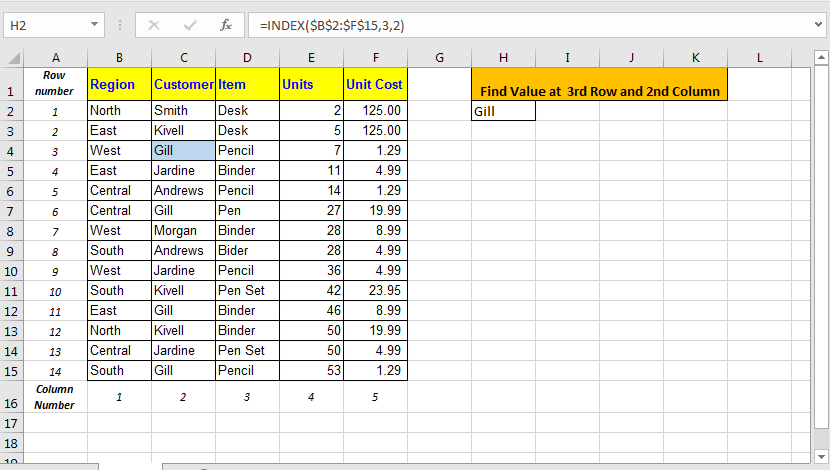Excel’s INDEX function has two forms known as:

1. Array Form INDEX Function
2. Reference Form INDEX Function

Lets understand them one by one.

## Array Form INDEX Function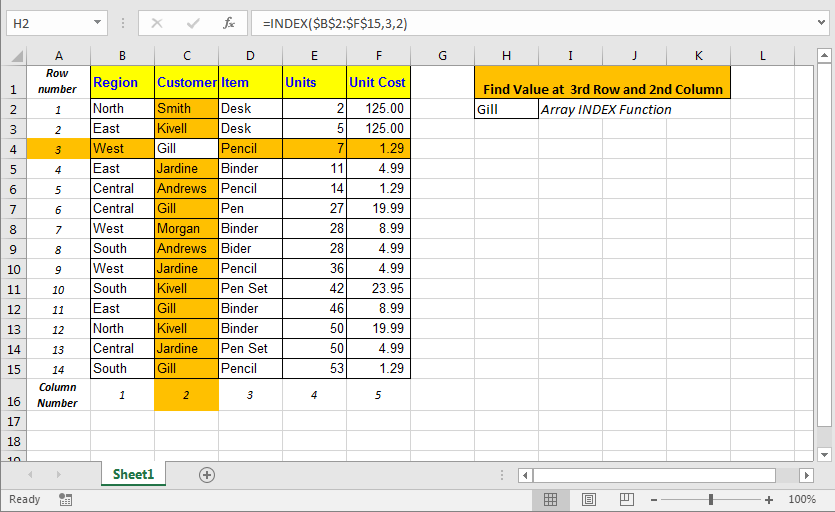Excel INDEX Function Array Form Syntax

=INDEX(array, row number, [optional column number]

Array : It is the range or an array.
Row number : Ther row number in your array from which you want to get your value.
[optional column number] : This column number in array. It is optional. If ommited INDEX formula automatically takes 1 for it.

Without anymore boring theory of INDEX function lets jump into an scenario.
I have this data.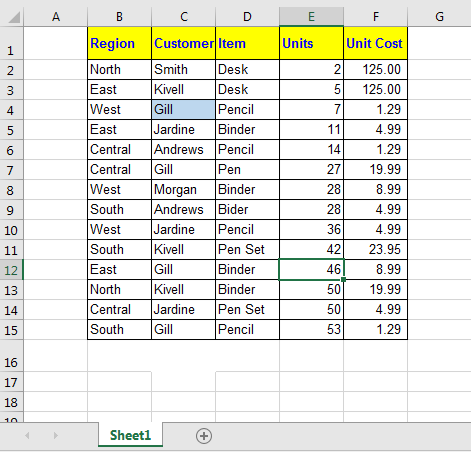I want to retrieve data at the intersection of 3rd Row and 2nd Column. I write this INDEX formula in cell H2:

=INDEX(\$B\$2:\$F\$15,3,2)

## Reference Form INDEX Function

It is much like multidimensional array index function. Actually in this form of INDEX function, we can give multiple arrays and then in the end we can tell index from which array to pull data.## Excel INDEX Function Reference Form Syntax

=INDEX( (array1, array2,...), row number, [optional column number], [optional array number] )

(array1, array2,...) : This parenthesis contains list of arrays. For example (A1:A10,D1:R100,...).
Row number : Ther row number in your array from which you want to get your value.
[optional column number] : This column number in array. It is optional. If omitted INDEX formula automatically takes 1 for it.
[optional array number] : The area number from which you want to pull data. In excel it is shown as area_num

Come, let’s have an example.

I have these 3 tables in Excel Worksheet.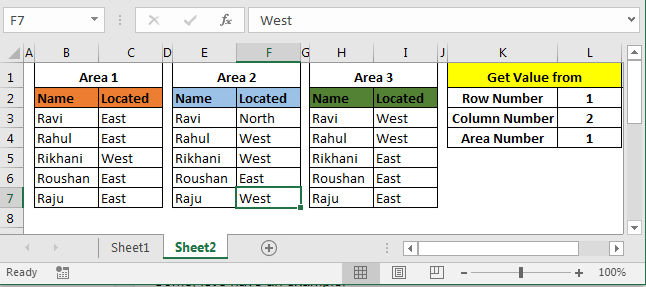Area 1, Area 2 and Area 3 are my ranges as shown in above image. I need to retrieve data according to values in cell L2, L3 and L4. So, I write this INDEX formula in cell M1.

=INDEX((\$B\$3:\$C\$7,\$E\$3:\$F\$7,\$H\$3:\$I\$7),L2,L3,L4)

Here L2 is 1, L3 is 2 and L4 is 1. Hence INDEX function will return value from 1st row of second column from 1st array. And that is East.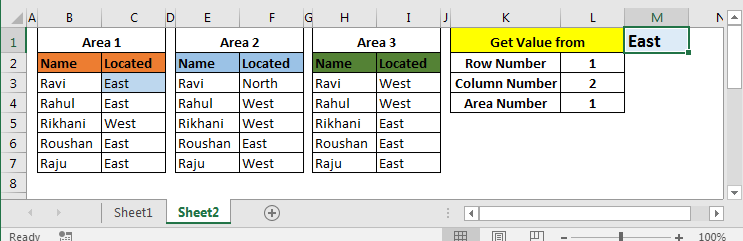Now change L2 to 2 and L4 to 2. You will have West in M2, as shown in below image.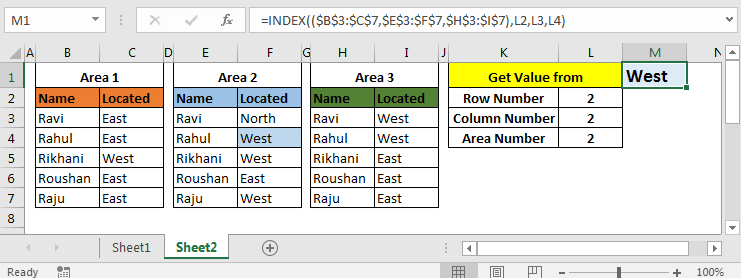And so on.

The INDEX function in Excel is mostly used with MATCH Function. The INDEX MATCH function is so famous that it is sometimes thought as one single function. Here I have explained INDEX MATCH function in detail. Go check it out.